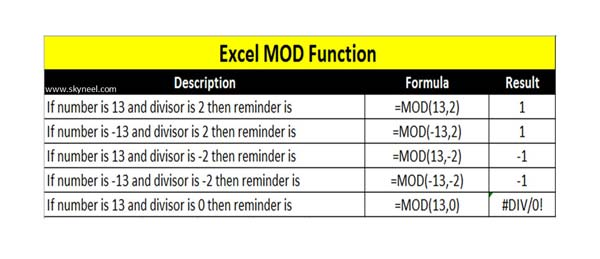# How to Use the Excel MOD Function to Get the Reminder

Excel MOD Function is located in Math and Trig category which allows you to quickly returns the remainder of two numbers after the division.

The Microsoft Excel MOD function is an important function of Excel, which is located in the Math and Trig category. The Microsoft Excel Mod function is used to returns the remainder after a number is divided by a divisor. The result has the same sign as division.

The MOD function is a built-in function of Excel. You can use this function as a worksheet function (WS) in the Microsoft Excel. The Excel MOD function is very popular and important function which quickly returns the remainder of two numbers after the division.

Most of the person want to know why MOD function is used in Excel? The answer of this query is that with the help of MOD function you can get the reminder after the division in two numbers. MOD function returns the numeric value as a result.

Syntax of MOD function in Excel

MOD (number, divisor)

Arguments: The MOD function has two arguments number, divisor.

number – It is an required argument. This is the number which you want to find the remainder. In other words, this number to be divided.

divisor – The second argument also required. This is the number by which you want to divide the number.

If you want to try to divide any number with 0 then you will get an error message #DIV/0! in active cell.

## How to use Excel MOD Function

This article helps you to understand about the MOD function. You have to take following steps in active worksheet to get the reminder.• If you want to get the reminder when number is 13 while divisor is 2 then you have to apply the given function =MOD(13,2) and press enter to get 1 as a result.
• If you have a negative number -13 while divisor is 2. In that situation you have to apply the given function =MOD(-13,2) and press enter to get 1 as a result.
• If both number and divisor are negative then you have to apply the given function
=MOD(-13,-2) and press enter to get -1 as a result.
• If number is positive and divisor is negative then you have to apply the given function
=MOD(13,-2) and press enter to get -1 as a result.
• If you try to divide any number with 0 divisor then you will get an error message
=MOD(13,0) and press enter to get #DIV/0! error message.

I hope after reading this guide you can easily use the MOD function to get the reminder. If you have any suggestion regarding this guide then please write us your suggestion in the comment box. Thanks to all.